Welcome to the Onshape forum! Ask questions and join in the discussions about everything Onshape.

First time visiting? Here are some places to start:
1. Looking for a certain topic? Check out the categories filter or use Search (upper right).
2. Need support? Ask a question to our Community Support category.
3. Please submit support tickets for bugs but you can request improvements in the Product Feedback category.
4. Be respectful, on topic and if you see a problem, Flag it.

If you would like to contact our Community Manager personally, feel free to send a private message or an email.

# how to copy bodies in each vector of a query?

I want to repeat a body in each value of a query I am creating, and then boolean all together, any easy way to do it?
I know there is opPattern but I dont get how to use it. I read the description from here https://cad.onshape.com/FsDoc/library.html#opPattern-Context-Id-map even then I don't get how to use it.
also to create the query I am using points= append(points, pt) and the function is inside a loop with a conditional (if true) stock pt in points, (if false) don't stock it. and when I add the append the FS becomes suuuper slow (not something that is impossible to use) I was asking myself if there is another way to do it.

for (var ix = -round(boxSize / blockSize, 1)-blockSize/millimeter; ix < (round(boxSize / blockSize, 1)+blockSize/millimeter); ix += 1) {
const x = 0 *millimeter+ (ix + 0.5) * blockSize;
for (var iy = -round(boxSize / blockSize,1)-blockSize/millimeter; iy < (round(boxSize / blockSize,1)+blockSize/millimeter); iy += 1) {
const y = 0*millimeter + (iy + 0.5) * blockSize;
for (var iz = -round(boxSize / blockSize,1)-blockSize/millimeter; iz < (round(boxSize / blockSize,1)+blockSize/millimeter); iz += 1) {
const z = 0*millimeter + (iz + 0.5) * blockSize;
const pt = vector(x, y, z);
if (size(evaluateQuery(context, qContainsPoint(definition.bodies, pt))) > 0)
{points= append(points, pt);
}
}
}
}
Tagged:

## Comments

• Moderator, Onshape Employees Posts: 3,691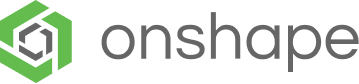edited September 2019
How big are the x, y and z loops? Is it generating dozens or thousands of points? That would explain why it is slow. It is not doing any geometry calculations - the slowest line on there is the append - it has to make a new sized array and copy the contents from the old one each time. What is the end goal?
Director, Technical Services, EMEAI
• Moderator, Onshape Employees Posts: 3,691edited September 2019
Try calculating the maximum array size first (x * y * z) then use makeArray. Assuming the points can be in any order, you could then just use and increment a counter:
```points[count] = pt;
count +=1;```
See if that is any faster. At the end you could resize the array based on "count".
Director, Technical Services, EMEAI
• NeilCooke said:
How big are the x, y and z loops? Is it generating dozens or thousands of points? That would explain why it is slow. It is not doing any geometry calculations - the slowest line on there is the append - it has to make a new sized array and copy the contents from the old one each time. What is the end goal?
the end goal, is to create a FS that will take a body, and create the same body but with a blockish look something like this: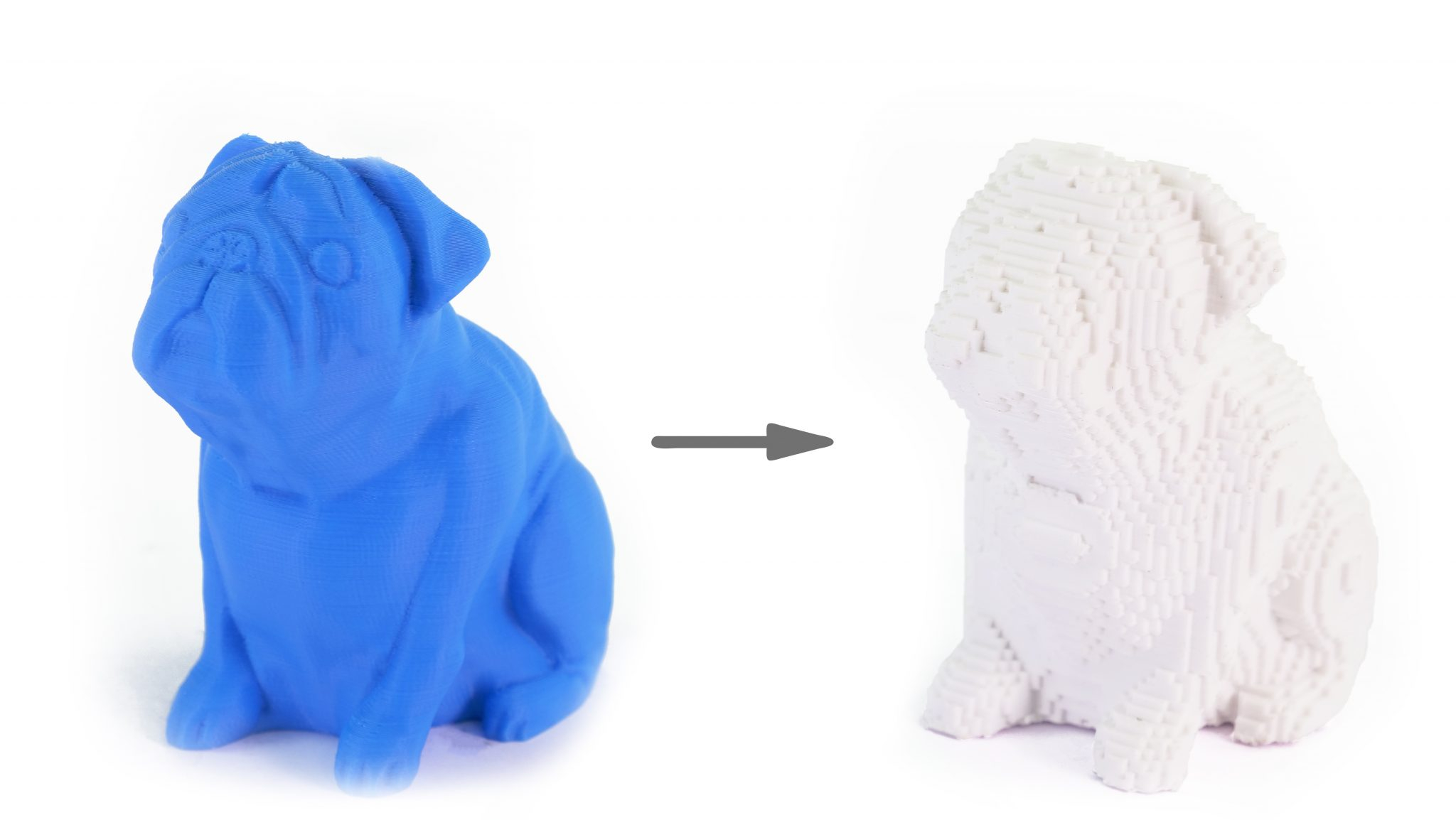the idea is to be able to put the X',Y',Z' directions of the cubes not necessarily in the same x,y,z of the original body, what I am doing is:
turning original body for the new XYZ'.
creating a minimum box that contains the turned body
doing 3 loops to make the grid of points, and keep only the ones that are inside of the body ( if (size(evaluateQuery(context, qContainsPoint(definition.bodies, pt))) > 0))
then I stock the points inside a query
copy the "block" in each point of the query
intersect the bodies with the original one
re turning the final body to original XYZ.
thats my goal, from my goal to achive it is another thing ahahaha. but I have at least for the moment with the debug successfully show the box that contains the turned body, and the inside points.
• Member, OS Professional, Developers Posts: 1,948 EDU
@Otaola_Franco

Here is an example feature that does what you want.

```FeatureScript 1150;
import(path : "onshape/std/geometry.fs", version : "1150.0");

annotation { "Feature Type Name" : "Voxelize" }
export const voxelize = defineFeature(function(context is Context, id is Id, definition is map)
precondition
{
annotation { "Name" : "Bodies", "Filter" : EntityType.BODY && BodyType.SOLID && AllowMeshGeometry.YES }
definition.entities is Query;

annotation { "Name" : "Voxel size" }
isLength(definition.voxelSize, BLEND_BOUNDS);

annotation { "Name" : "Build voxels" }
definition.buildVoxels is boolean;
}
{
var bodies = qUnion(evaluateQuery(context, definition.entities));
var voxelSize = definition.voxelSize;
var buildVoxels = definition.buildVoxels;
var boundingBox = evBox3d(context, {
"topology" : bodies,
"tight" : false // It doesn't matter if the box is a slight bit too large
});
var bounds = {
"minCorner" : mapArray(boundingBox.minCorner, function(value)
{
return floor(value / voxelSize);
}) as Vector,
"maxCorner" : mapArray(boundingBox.maxCorner, function(value)
{
return ceil(value / voxelSize);
}) as Vector
};
var out = makeArray(bounds.maxCorner - bounds.minCorner, makeArray(bounds.maxCorner - bounds.minCorner, makeArray(bounds.maxCorner - bounds.minCorner, undefined)));
for (var x = bounds.minCorner + 0.5; x < bounds.maxCorner; x += 1)
for (var y = bounds.minCorner + 0.5; y < bounds.maxCorner; y += 1)
for (var z = bounds.minCorner + 0.5; z < bounds.maxCorner; z += 1)
{
var point = vector(x, y, z);
var pointVWU = point * voxelSize;
if (evaluateQuery(context, qContainsPoint(bodies, pointVWU)) != [])
out[x - (bounds.minCorner + 0.5)][y - (bounds.minCorner + 0.5)][z - (bounds.minCorner + 0.5)] = point;
}
// Debug the points.
// Something else could be done herefor (var xSlice in out)
for (var xySlice in xSlice)
for (var point in xySlice)
if (point != undefined)
debug(context, point * voxelSize);
});
```

MB - I make FeatureScripts: View FeatureScripts
• @Otaola_Franco

Here is an example feature that does what you want.

```FeatureScript 1150;
import(path : "onshape/std/geometry.fs", version : "1150.0");

annotation { "Feature Type Name" : "Voxelize" }
export const voxelize = defineFeature(function(context is Context, id is Id, definition is map)
precondition
{
annotation { "Name" : "Bodies", "Filter" : EntityType.BODY && BodyType.SOLID && AllowMeshGeometry.YES }
definition.entities is Query;

annotation { "Name" : "Voxel size" }
isLength(definition.voxelSize, BLEND_BOUNDS);

annotation { "Name" : "Build voxels" }
definition.buildVoxels is boolean;
}
{
var bodies = qUnion(evaluateQuery(context, definition.entities));
var voxelSize = definition.voxelSize;
var buildVoxels = definition.buildVoxels;
var boundingBox = evBox3d(context, {
"topology" : bodies,
"tight" : false // It doesn't matter if the box is a slight bit too large
});
var bounds = {
"minCorner" : mapArray(boundingBox.minCorner, function(value)
{
return floor(value / voxelSize);
}) as Vector,
"maxCorner" : mapArray(boundingBox.maxCorner, function(value)
{
return ceil(value / voxelSize);
}) as Vector
};
var out = makeArray(bounds.maxCorner - bounds.minCorner, makeArray(bounds.maxCorner - bounds.minCorner, makeArray(bounds.maxCorner - bounds.minCorner, undefined)));
for (var x = bounds.minCorner + 0.5; x < bounds.maxCorner; x += 1)
for (var y = bounds.minCorner + 0.5; y < bounds.maxCorner; y += 1)
for (var z = bounds.minCorner + 0.5; z < bounds.maxCorner; z += 1)
{
var point = vector(x, y, z);
var pointVWU = point * voxelSize;
if (evaluateQuery(context, qContainsPoint(bodies, pointVWU)) != [])
out[x - (bounds.minCorner + 0.5)][y - (bounds.minCorner + 0.5)][z - (bounds.minCorner + 0.5)] = point;
}
// Debug the points.
// Something else could be done herefor (var xSlice in out)
for (var xySlice in xSlice)
for (var point in xySlice)
if (point != undefined)
debug(context, point * voxelSize);
});
```

hi bartlett from what i saw in your feature it does the same as where I was (more effectively i can say....) but I find myself at the same point, your code only shows the points inside the body but i still have the problem i dont get how to pattern the body in the vectors.
• Moderator, Onshape Employees Posts: 3,691edited September 2019
Slightly modified script with opPattern:

```FeatureScript 1150;
import(path : "onshape/std/geometry.fs", version : "1150.0");

annotation { "Feature Type Name" : "Voxelize 2" }
export const voxelize = defineFeature(function(context is Context, id is Id, definition is map)
precondition
{
annotation { "Name" : "Bodies", "Filter" : EntityType.BODY &amp;&amp; BodyType.SOLID &amp;&amp; AllowMeshGeometry.YES }
definition.entities is Query;

annotation { "Name" : "Voxel size" }
isLength(definition.voxelSize, BLEND_BOUNDS);

annotation { "Name" : "Build voxels" }
definition.buildVoxels is boolean;
}
{
var bodies = qUnion(evaluateQuery(context, definition.entities));
var voxelSize = definition.voxelSize;
var buildVoxels = definition.buildVoxels;

fCuboid(context, id + "cuboid", {
"corner1" : vector(-0.5, -0.5, -0.5) * voxelSize * 1.01, // to make sure voxels overlap when adding boolean later
"corner2" : vector(0.5, 0.5, 0.5) * voxelSize * 1.01
});

var boundingBox = evBox3d(context, {
"topology" : bodies,
"tight" : false // It doesn't matter if the box is a slight bit too large
});

var bounds = {
"minCorner" : mapArray(boundingBox.minCorner, function(value)
{
return floor(value / voxelSize);
}) as Vector,
"maxCorner" : mapArray(boundingBox.maxCorner, function(value)
{
return ceil(value / voxelSize);
}) as Vector
};
var maxArraySize = (bounds.maxCorner - bounds.minCorner) * (bounds.maxCorner - bounds.minCorner) * (bounds.maxCorner - bounds.minCorner);
var points = makeArray(maxArraySize);
var transforms = makeArray(maxArraySize);
var instanceNames = makeArray(maxArraySize);
var count = 0;
for (var x = bounds.minCorner + 0.5; x &lt; bounds.maxCorner; x += 1)
for (var y = bounds.minCorner + 0.5; y &lt; bounds.maxCorner; y += 1)
for (var z = bounds.minCorner + 0.5; z &lt; bounds.maxCorner; z += 1)
{
var point = vector(x, y, z) * voxelSize;
if (evaluateQuery(context, qContainsPoint(bodies, point)) != [])
{
points[count] = point;
transforms[count] = transform(point);
instanceNames[count] = toString(count);
count += 1;
}
}

points = resize(points, count);
transforms = resize(transforms, count);
instanceNames = resize(instanceNames, count);

for (var point in points)
{
debug(context, point);
}

if (definition.buildVoxels)
{
opPattern(context, id + "pattern", {
"entities" : qCreatedBy(id + "cuboid", EntityType.BODY),
"transforms" : transforms,
"instanceNames" : instanceNames
});

opDeleteBodies(context, id + "deleteBodies", {
"entities" : qCreatedBy(id + "cuboid", EntityType.BODY)
});

opBoolean(context, id + "boolean", {
"tools" : qCreatedBy(id + "pattern", EntityType.BODY),
"operationType" : BooleanOperationType.UNION
});
}
});```

Director, Technical Services, EMEAI
• Member, OS Professional, Developers Posts: 1,948 EDU
@NeilCooke

If you add `opOffsetFace(context, id + "offsetIn", { "faces" : qCreatedBy(id, EntityType.FACE), "offset" : -voxelSize * 0.005 })` at the end, it will fix the extra size of the voxels
MB - I make FeatureScripts: View FeatureScripts
• NeilCooke said:
Slightly modified script with opPattern:

```FeatureScript 1150;
import(path : "onshape/std/geometry.fs", version : "1150.0");

annotation { "Feature Type Name" : "Voxelize 2" }
export const voxelize = defineFeature(function(context is Context, id is Id, definition is map)
precondition
{
annotation { "Name" : "Bodies", "Filter" : EntityType.BODY &amp;&amp; BodyType.SOLID &amp;&amp; AllowMeshGeometry.YES }
definition.entities is Query;

annotation { "Name" : "Voxel size" }
isLength(definition.voxelSize, BLEND_BOUNDS);

annotation { "Name" : "Build voxels" }
definition.buildVoxels is boolean;
}
{
var bodies = qUnion(evaluateQuery(context, definition.entities));
var voxelSize = definition.voxelSize;
var buildVoxels = definition.buildVoxels;

fCuboid(context, id + "cuboid", {
"corner1" : vector(-0.5, -0.5, -0.5) * voxelSize * 1.01, // to make sure voxels overlap when adding boolean later
"corner2" : vector(0.5, 0.5, 0.5) * voxelSize * 1.01
});

var boundingBox = evBox3d(context, {
"topology" : bodies,
"tight" : false // It doesn't matter if the box is a slight bit too large
});

var bounds = {
"minCorner" : mapArray(boundingBox.minCorner, function(value)
{
return floor(value / voxelSize);
}) as Vector,
"maxCorner" : mapArray(boundingBox.maxCorner, function(value)
{
return ceil(value / voxelSize);
}) as Vector
};
var maxArraySize = (bounds.maxCorner - bounds.minCorner) * (bounds.maxCorner - bounds.minCorner) * (bounds.maxCorner - bounds.minCorner);
var points = makeArray(maxArraySize);
var transforms = makeArray(maxArraySize);
var instanceNames = makeArray(maxArraySize);
var count = 0;
for (var x = bounds.minCorner + 0.5; x &lt; bounds.maxCorner; x += 1)
for (var y = bounds.minCorner + 0.5; y &lt; bounds.maxCorner; y += 1)
for (var z = bounds.minCorner + 0.5; z &lt; bounds.maxCorner; z += 1)
{
var point = vector(x, y, z) * voxelSize;
if (evaluateQuery(context, qContainsPoint(bodies, point)) != [])
{
points[count] = point;
transforms[count] = transform(point);
instanceNames[count] = toString(count);
count += 1;
}
}

points = resize(points, count);
transforms = resize(transforms, count);
instanceNames = resize(instanceNames, count);

for (var point in points)
{
debug(context, point);
}

if (definition.buildVoxels)
{
opPattern(context, id + "pattern", {
"entities" : qCreatedBy(id + "cuboid", EntityType.BODY),
"transforms" : transforms,
"instanceNames" : instanceNames
});

opDeleteBodies(context, id + "deleteBodies", {
"entities" : qCreatedBy(id + "cuboid", EntityType.BODY)
});

opBoolean(context, id + "boolean", {
"tools" : qCreatedBy(id + "pattern", EntityType.BODY),
"operationType" : BooleanOperationType.UNION
});
}
});```

hello neil and mbartlett,
first of all, thanks for the GREAT help.
I have some questions about the scripts:
1. if the body is slightly bigger than half of the cell size, the voxelized body would not contain all the original body, how could I do to add an extra line in each direction so the howl original body can be contained inside? to show what I meant in the next fig I colored (in red) the parts that stay out of the voxelized body (original a cylinder)  (voxelized in transparent blue and in green the body that is inside the voxelized created body)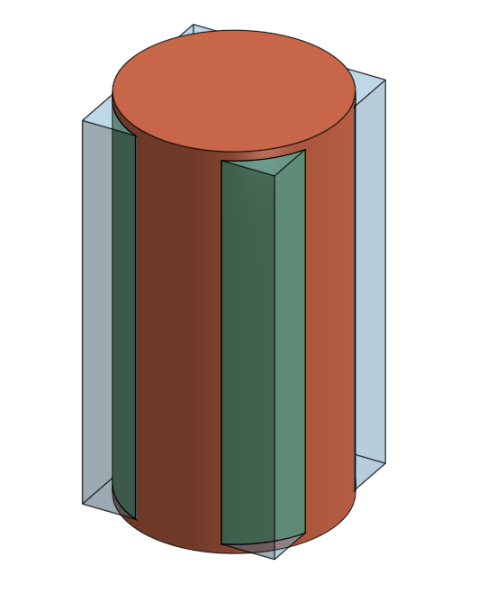2.if I understand correctly instancename (line 45,56) is necessary for opPattern(line 71), (but what is inside it is not important as it is the name of each produced body for the oppattern). wouldnt be more efficient to create it ( and also transforms inline 44 and 55) after the loop and the resize of points? so it would be faster?

3. I don't get how transforms works, I see that inside has transform so I assume that is to each time move the object patterned to the right position, i am correct? so it is an array composed of functions? (each time transform)

4. I saw that inline 23 to be sure that is going to boolean you add a little bit of extra in the sizes I am planning to use another body that is contained inside the cube and modify the FS to be capable of select it and use it in the pattern, should I add some extra in the body to assure this too? (I don't get exactly why as the points were created using the block size... I thought that maybe it was easier for onshape to boolean bodies in union that had intersected volume more than intersecting surfaces, is that the case?)

5.from the comment of mbartlett opOffsetFace(context, id + "offsetIn", { "faces" : qCreatedBy(id, EntityType.FACE), "offset" : -voxelSize * 0.005 }) would eliminate the extra 0.01 of the voxel size that was created for the situation in question 4? is that?

6.when I finish doing the FS I would like to publish as an EDU FS but I couldn't find how to publish something with an EDU status, how can I do this?

also, thanks for all the help guys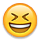.
• Moderator, Onshape Employees Posts: 3,691Here goes:
1. Because it is voxelized it is an approximation. If you tried to check each voxel to see if it fully enclosed the solid the script would grind to a halt (very expensive in compute terms). You would have to use a smaller voxel size.

2. Unlikely or possibly only marginally.

3. It is an array of transforms, one for each instance - the original voxel is created at the origin so the point is all that's needed.

4. If the blocks touch at edges or vertices the boolean will fail so it's best to have an overlap

5. Correct

6. Not sure what you mean by EDU FS? Do you have an EDU account? All FS are just public.
Director, Technical Services, EMEAI
• NeilCooke said:

All FS are just public.
Presuming they're in a public document in the first place?
Business Systems and Configuration Controller
HWM-Water Ltd
• NeilCooke said:
Here goes:
1. Because it is voxelized it is an approximation. If you tried to check each voxel to see if it fully enclosed the solid the script would grind to a halt (very expensive in compute terms). You would have to use a smaller voxel size.

2. Unlikely or possibly only marginally.

3. It is an array of transforms, one for each instance - the original voxel is created at the origin so the point is all that's needed.

4. If the blocks touch at edges or vertices the boolean will fail so it's best to have an overlap

5. Correct

6. Not sure what you mean by EDU FS? Do you have an EDU account? All FS are just public.
1. yeah but I am looking that "at least" contains all the object, so if it is a little bit bigger wouldn't be a problem. the only way I see a solution for this is to:
calculate the remainder of each size of the boundingBox and the size of the cube (remainder x, remainder y, remainder z)
if the remainder i < size i of the cube (so there is a smaller part than half of the cube size, in the positive and in the negative direction)
then scale the original body in that direction i by the ((cube size+boundingBox size i) /boundingBox size i)
change the bounds with a new boundingBox of the scaled body
use the new scaled body in (evaluateQuery(context, qContainsPoint(bodies, point)) != [])
that way the voxelize would be sligly bigger than the orignal body.
what you think? is the only way i can thing to extend the list of points by one block in each direction if it is not completely contained.
2. okey, going to give a try, but yeah, i can see that would be marginally but as the howl process is machine consuming I am going to try to optimize it the better i can.

3.excalty what I was understanding, god FS language is powerful.

4. but they don't touch by edges or vertices only, they touch by surfaces, no?

5. okis6. an educational document I was trying to s

thanks
• NeilCooke said:

All FS are just public.
Presuming they're in a public document in the first place?
would love to see spotlights of not public FS only descriptions not the code obviously, but I would say that a looot of really good users are pro ones, it could be cool to see what they have done (even if the FS itself is not shared)
• Moderator, Onshape Employees, Developers Posts: 1,636@Otaola_Franco

Imagine a scenario where this is the solution: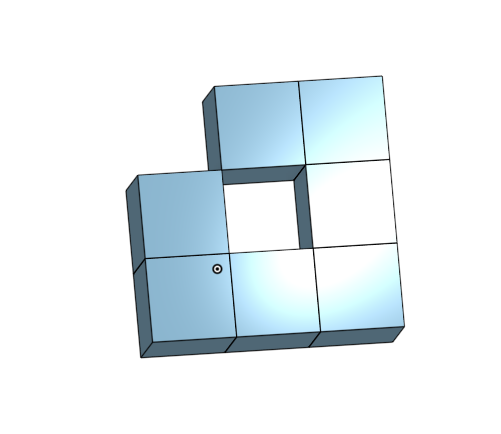A boolean will fail on that because if that thing was one solid body, it would have an edge that bounds 4 faces.

You can imagine a slightly more complex similar scenario for a shared non-manifold vertex.

Making the cubes slightly larger solves this.
Jake Rosenfeld - Modeling Team
• Moderator, Onshape Employees, Developers Posts: 1,636edited September 2019
For #6, EDU docs are docs created by EDU users.  If you are a student, you can get an EDU plan.  Here are some details: https://www.onshape.com/products/education

For #1, for something that is a little more robust but still not technically correct for all cases, try the following:  Imagine the grid of cubes you have already set up.  Instead of testing at the center of each cube, test at the center of each cube face.  If there is material at the center of the cube face, then the two cubes sharing this face should both be constructed.  If you are careful, this algorithm will not need to be for (each cube) { for (each face center) {} } because each face center actually belongs to two cubes.  What I would do is something along the lines of the top, right, and back face of each cube, and then add then add both cubes when you get a hit.

If you use this solution, you should also optimize by not adding a transform to the transforms array if it is already in there.  If you do that, you'll get lots of extra geometry that will just slow the system down.

Some psuedocode:

```{
...

.  var cubesAlreadyAdded = [];
for (i)
{
for (j)
{
for (k)
{
if (right face center is a hit)
{
if (!hasCubeBeenAdded[i][j][k])
{
cubesAlreadyAdded = addCube(cubesAlreadyAdded, i, j, k);
// Do other work to add cubes to transforms, etc.
}
if (!hasCubeBeenAdded[i + 1][j][k])
{
cubesAlreadyAdded = addCube(cubesAlreadyAdded, i + 1, j, k);
// Do other work to add cubes to transforms, etc.
}
}
if (back face center is a hit)
{
// Same as right face center, but do it for this cube and for [i][j + 1][k]
}
if (top face center is a hit)
{
// Same as right face center, but do it for this cube and for [i][j][k + 1]
}
}
}
}

...
}

function hasCubeBeenAdded(cubesAlreadyAdded is map, i is number, j is number, k is number) returns boolean
{
if (cubesAlreadyAdded[i] == undefined)
return false;
if (cubesAlreadyAdded[i][j] == undefined)
return false;
if (cubesAlreadyAdded[i][j][k] == undefined)
return false;
return true;
}

function addCube(cubesAlreadyAdded is map, i is number, j is number, k is number) returns map
{
if (cubesAlreadyAdded[i] == undefined)
cubesAlreadyAdded[i] = {};
if (cubesAlreadyAdded[i][j] == undefined)
cubesAlreadyAdded[i][j] = {};
if (cubesAlreadyAdded[i][j][k] == undefined)
cubesAlreadyAdded[i][j][k] = true;
return cubesAlreadyAdded;
}

```

Jake Rosenfeld - Modeling Team
• For #6, EDU docs are docs created by EDU users.  If you are a student, you can get an EDU plan.  Here are some details: https://www.onshape.com/products/education

For #1, for something that is a little more robust but still not technically correct for all cases, try the following:  Imagine the grid of cubes you have already set up.  Instead of testing at the center of each cube, test at the center of each cube face.  If there is material at the center of the cube face, then the two cubes sharing this face should both be constructed.  If you are careful, this algorithm will not need to be for (each cube) { for (each face center) {} } because each face center actually belongs to two cubes.  What I would do is something along the lines of the top, right, and back face of each cube, and then add then add both cubes when you get a hit.

If you use this solution, you should also optimize by not adding a transform to the transforms array if it is already in there.  If you do that, you'll get lots of extra geometry that will just slow the system down.

Some psuedocode:

```{
...

.  var cubesAlreadyAdded = [];
for (i)
{
for (j)
{
for (k)
{
if (right face center is a hit)
{
if (!hasCubeBeenAdded[i][j][k])
{
cubesAlreadyAdded = addCube(cubesAlreadyAdded, i, j, k);
// Do other work to add cubes to transforms, etc.
}
if (!hasCubeBeenAdded[i + 1][j][k])
{
cubesAlreadyAdded = addCube(cubesAlreadyAdded, i + 1, j, k);
// Do other work to add cubes to transforms, etc.
}
}
if (back face center is a hit)
{
// Same as right face center, but do it for this cube and for [i][j + 1][k]
}
if (top face center is a hit)
{
// Same as right face center, but do it for this cube and for [i][j][k + 1]
}
}
}
}

...
}

function hasCubeBeenAdded(cubesAlreadyAdded is map, i is number, j is number, k is number) returns boolean
{
if (cubesAlreadyAdded[i] == undefined)
return false;
if (cubesAlreadyAdded[i][j] == undefined)
return false;
if (cubesAlreadyAdded[i][j][k] == undefined)
return false;
return true;
}

function addCube(cubesAlreadyAdded is map, i is number, j is number, k is number) returns map
{
if (cubesAlreadyAdded[i] == undefined)
cubesAlreadyAdded[i] = {};
if (cubesAlreadyAdded[i][j] == undefined)
cubesAlreadyAdded[i][j] = {};
if (cubesAlreadyAdded[i][j][k] == undefined)
cubesAlreadyAdded[i][j][k] = true;
return cubesAlreadyAdded;
}

```

hi jake!

thanks for the clarification with educational vr, I thought that in a public accound you could also public educational. i am going to look in the edu plan, thanks.
for your fist comment, ups i didnt though of a geometry like that ahahahaha, but you are right, thanksfor your second message, i am sorry it is super late and a little bit tired (and preeety sure that i am wrong....) but, is it always true? i though of an example like this: (depending on the size of the cell it would or not be true...)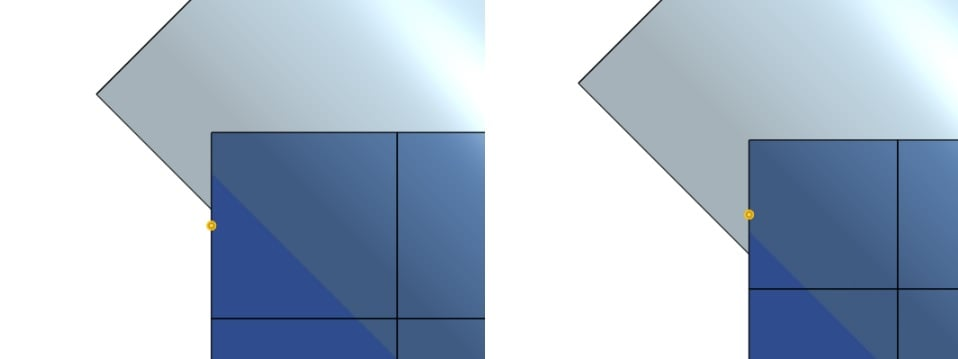also another question, as i mentioned before, the final idea is to have a contructed cell that i am going to be able to choose for the moment,as a second body in the part studio, for this i modified the code and add an entry for a body
annotation { "Name" : "cell", "Filter" : EntityType.BODY }
definition.cell is Query;

and after i changed in the opPattern opPattern(context, id + "pattern", { "entities" : qCreatedBy(id + "cell", EntityType.BODY), "transforms" : transforms, "instanceNames" : instanceNames }); but it not working, i know the error is inside the last if as when i turn definition.buildVoxels false the FS works, (and is not the cell geometry the problem as i even tried a cube as the one created from the fcuboid in the script.
• Moderator, Onshape Employees, Developers Posts: 1,636@Otaola_Franco

In the picture you provided, the block to the left of the yellow dot may or may not be built.  It won't be built off the cube to the right, as you have shown.  But it might be built from the top depending on where that midpoint is: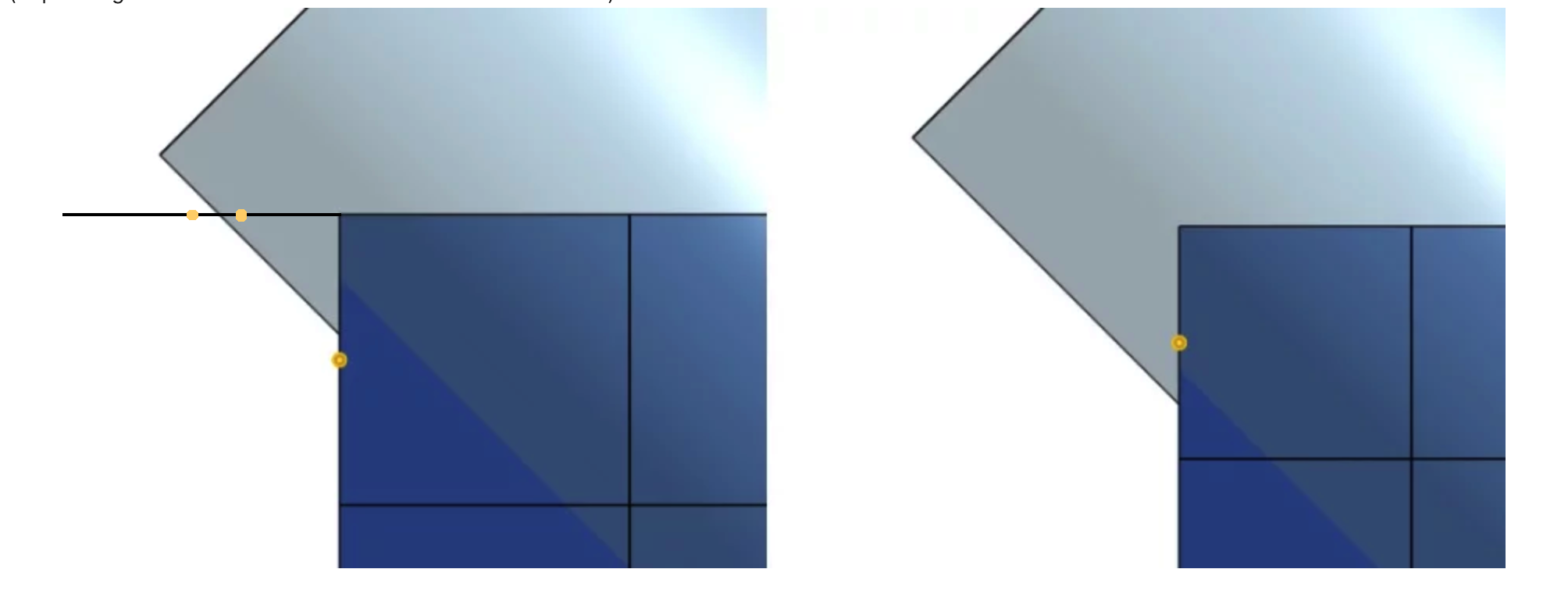like I said though, this method won't be perfect either, it will just be better than using the centers.

If you want it perfect, you could use `evDistance` to find the closest point to the center, and see if that point is inside the cube, but that will be super slow.  Maybe some kind of `evDistance` approach combined with spatial division using an oct-tree?  https://en.wikipedia.org/wiki/Octree

Depending on how much work you want to do you can go down a major rabbit hole here.

For you second question, you want

`"entities" : definition.cell`
not
`"entities" : qCreatedBy(id + "cell", EntityType.BODY)`

Jake Rosenfeld - Modeling Team
• edited September 2019
@Otaola_Franco

In the picture you provided, the block to the left of the yellow dot may or may not be built.  It won't be built off the cube to the right, as you have shown.  But it might be built from the top depending on where that midpoint is:like I said though, this method won't be perfect either, it will just be better than using the centers.

If you want it perfect, you could use `evDistance` to find the closest point to the center, and see if that point is inside the cube, but that will be super slow.  Maybe some kind of `evDistance` approach combined with spatial division using an oct-tree?  https://en.wikipedia.org/wiki/Octree

Depending on how much work you want to do you can go down a major rabbit hole here.

For you second question, you want

`"entities" : definition.cell`
not
`"entities" : qCreatedBy(id + "cell", EntityType.BODY)`

lol, i feel in the rabbit howl already ahahahaha, never thought that I would be trying to develop a FS. (from one year before that i was using Onshape for the first time and even first time CAD in general.... )
well, I find myself with an issue, when I use another "seed" instead of the cube, now the document gives me the error (ab0f9fef133a4db30ed79d2a), I know that is because it is too complex as when I resized the same seed but bigger it worked correctly, is there any way around this? I mean by an API or aaany way that I can export the result in a.STEP or other file types where I can have the final part?
thanks for all the help guys, the onshape team is always surprising me.

maybe you would like the link of the document:https://cad.onshape.com/documents/868b98813dc2470facff8624/w/7aaae9fc38becda0507096c6/e/5fc5e3ce45f6741d0263f932
Sign In or Register to comment.

#### Howdy, Stranger!

It looks like you're new here. If you want to get involved, click one of these buttons!